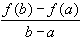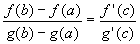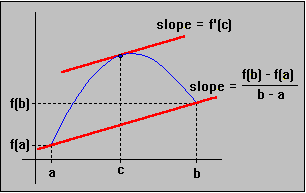#Interactive Real Analysis

Next | Previous | Glossary | Map

## 6.5. Differentiable Functions

### Theorem 6.5.9: Mean Value Theorem

If f is continuous on [a, b] and differentiable on (a, b), then there exists a number c in (a, b) such that
f'(c) =If f and g are continuous on [a, b] and differentiable on (a, b) and g'(x) # 0 in (a, b) then there exists a number c in (a, b) such that### Proof:

The first version of the Mean Value theorem is actually Rolle's theorem in disguise. A simple linear function can convert one situation into the other:geometric interpretation of MVT

We need a linear function (linear so that we can easily compute its derivative) that maps the line through the two points ( a, f(a) ) and ( b, f(b) ) to the points ( a, 0 ) and ( b, 0 ). If we subtract that map from the function we will be in a situation where we can apply Rolle's theorem.

To find the equation of such a line is easy:

• y - f(a) =( x - a )

Thus, we define the following function:

• h(x) = f(x) -( x - a ) - f(a)

Then h is differentiable in ( a, b ) with h(a) = h(b) = 0. Therefore, Rolle's theorem guaranties a number c between a and b such that h'(c) = 0. But then

• 0 = h'(c) = f'(c) -which is exactly what we had to show for the first part.

The second part is very similar. You can fill in the details yourself by considering the function

• h(x) = f(x) -( g(x) - g(a) ) - f(a)Next | Previous | Glossary | Map